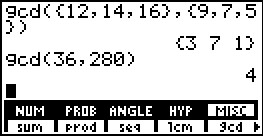# Knowledge Base

## Solution 18709: Calculating the gcd() (Greatest Common Divisor) on the TI-85 and TI-86 Graphing Calculators.

### How do I calculate the gcd() (Greatest Common Divisor) on the TI-85 and TI-86 graphing calculators?

The greatest common divisor (GCD) function returns the largest number that divides into two positive whole numbers or lists of positive whole numbers evenly. If both arguments are lists, they must have the same number of elements. If one argument is a list and the other a non-list, the non-list is paired with each element of the list, and a list is returned.

The syntax is as follows:

gcd(valueA,valueB)

gcd(listA,listB)

Examples:

• Find the greatest common divisor for lists A and B: {12,14,16}, {9,7,5}

• Find the greatest common divisor for 36 and 280.

The gcd() function can be located by following the keystrokes provided below:

• Press[2nd] [MATH].

• Press [F5] [F5] for gcd(.

• Input the values or lists separated by a [,] and press [)] [ENTER].

Please see the examples provided below: